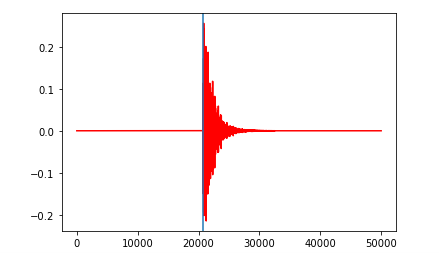# Hyperparameter tuning problem in time series regression

Hello everyone,
I have a time series regression problem. I want to predict a single value based on a time series.
To problem is to predict the arrival time of a signal. The blue line below is the label (arrival time) and the red is the signal. I have 2000 of these samples.

The time series has the length 50,000, I used tsai’s LSTM, 1D CNNs and other models, but I couldn’t get the desired results. The predicted value is always around a constant, which is kind of the average of the labels. I suspect that something is wrong with my choice of hyperparameters and loss function, can anyone suggest a solution?
My code is as follows:

from tsai.all import *
get_ipython().run_line_magic(‘matplotlib’, ‘inline’)

BS=10

X=Simulation_Data.reshape(2000, 1, -1)
y=Label.reshape(2000,)/25000

splits = (L(np.arange(int(len(y)*0.8)), use_list=True),
L(np.arange(int(len(y)*0.8), len(y)), use_list=True))

check_data(X, y, splits)

tfms = [None, [TSRegression()]]
batch_tfms = TSStandardize(by_sample=True, by_var=True)
dls = get_ts_dls(X, y, splits=splits, tfms=tfms, batch_tfms=batch_tfms, bs=BS) #TSDataLoaders.from_dsets
dls.one_batch()

model = LSTM(dls.vars, dls.c,hidden_size=10 ,bidirectional=True)

learn = ts_learner(dls, model,
metrics=[mae, rmse], cbs=ShowGraph()) #metrics=[f1]

learn.lr_find()

learn.fit_one_cycle(1, 1e-3)

learn.fit_one_cycle(20, slice(1e-8, (1e-2)/5))

learn.fit_one_cycle(20, slice(1e-8, 1e-3))

valid_preds, valid_targets = learn.get_preds(ds_idx=1)

valid_preds.flatten().data

valid_preds = valid_preds.cpu().detach().numpy()
valid_targets = valid_targets.cpu().detach().numpy()